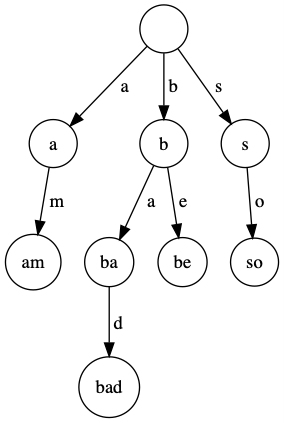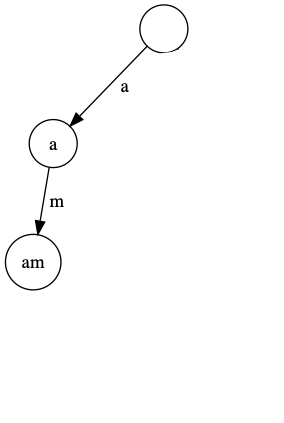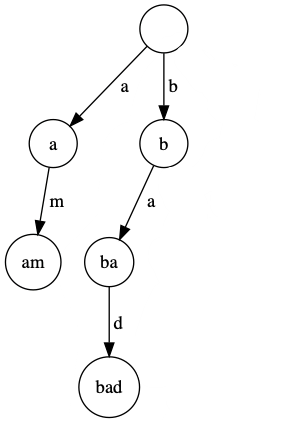Introduction to Tries ( Insert and Search )

Trie is a tree like data structure where we store string of words or alphabets and can be retrieved by traversing down the path of the tree. It consists of nodes and edges. Each edge connect each parent node to its children.

Trie data structure is very beneficial when a problem is based on prefixes of a string.

What is prefix of a string ?

Let us consider the string s=”abccfeg”

The prefixes of the string s are :

a

ab

abc

abcc

abccf

abccfe

abccfeg

Each Trie has an empty root node and all the nodes contain alphabets or strings connected by edges.

Tries are generally used on groups of multiple string rather than using a single string.

For example, Consider an English dictionary and a string s, find the prefix of the dictionary strings matching the string s. Solving this problem using a brute-force approach would require us to match the prefix of the given string with the prefix of every other word in the dictionary takes more run time. This is an inefficient and costly process. Using Tries, we can solve this problem in much more efficient way.Operations in Trie :

• Insert
• Search
• Delete

Let us see how the words are inserted into the Trie.

Let the words be { “am” , “bad” , “be” , “so” }

Initially Trie is empty.

Let us see how the string “am” is inserted into Trie.The next word “bad” is inserted into Trie.Inserting the word “be” into Trie.Inserting the word “so” into Trie.Let us see how to check whether the given word is present in the Trie or not.1. For the string “badd” we move from b -> a -> d after iterating d ,we see that the next letter ‘d’ is not present in the Trie. Hence it returns False.

2. For the string “am” we move from a -> m and at the letter “m” we find the end of word is 1. Hence we return True.

3. For string “s” we moved to s and at the letter “s” we find the end of word is 0.Hence,we return False.

4. For string “be” we move from b->e and at the letter “e” we find that end of word is 0.Hence,we return True.

Output :

0
1
0
1

For getting grip over Trie , Try solving these problems !

Happy Coding 😊

By Programmers Army

Contributed by: Ravi Teja Chidurala